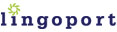# Locale-Sensitive Java Method

### `java.lang.Float`

`public static float parseFloat(String s)`

### Internationalization (I18n) Method Overview

The `parseFloat` method returns a `float` primitive value by parsing a floating point string.

### I18n Issues

The problem with calling `parseFloat` is not locale aware. For example, if a string is set to `"2,3"` where a comma is used as the decimal separator, the `parseFloat` method will not create the correct `float` primitive value. This is because it is designed to assume that a period will be used as the decimal separator.

### Suggested Replacement

To ensure that locale is applied when converting from float strings to float values, use the `DecimalFormat` class.

```float myFloat = Float.parseFloat(myFloatString); ```

Use:

``` float myFloat = 0; //Retrieve the runtime user's locale Locale locale = getUserLocale(); //Now call the NumberFormat factory method //and pass the locale object NumberFormat f = NumberFormat.getInstance(locale); //If it is in fact an instance of DecimalFormat, //cast it as such and use it as needed if (f instanceof DecimalFormat) {     try {         myFloat = ((DecimalFormat) f).parse(myFloatString).floatValue();     } catch (ParseException e) {} } ```